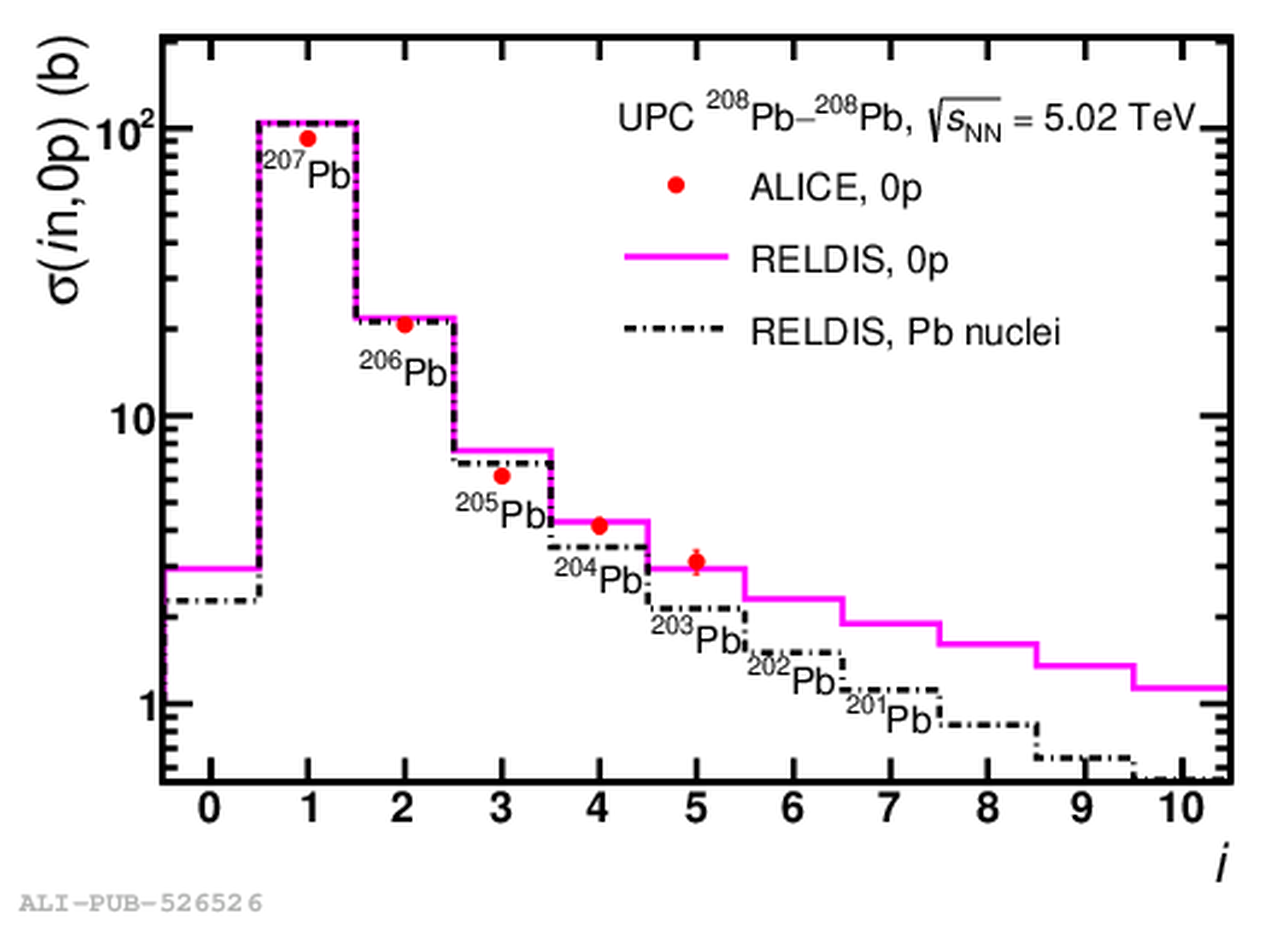# Figure 3

 Colour online: measured cross sections of emission of given numbers of neutrons $i$ in UPCs of $^{208}$Pb nuclei at $\sqrt{s_{\mathrm{NN}}}=5.02$ TeV without proton emission (points) and the cross sections calculated with RELDIS  (solid-line histogram). Calculated cross sections to produce specific secondary nuclei, $^{207,206,205,204,203,202,201}$Pb, are presented by the dash-dotted histogram marked by nuclide symbols. Combined statistical and systematic uncertainties of the measurements are presented..